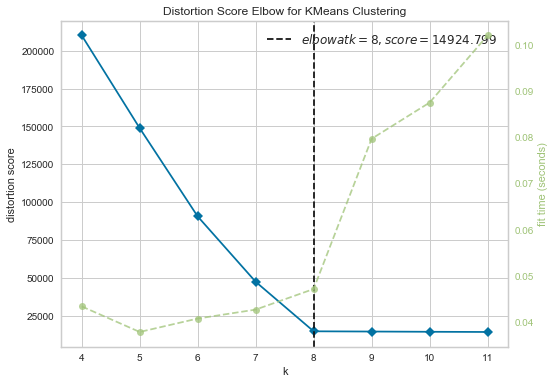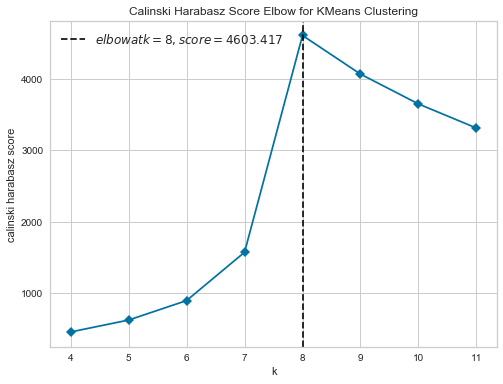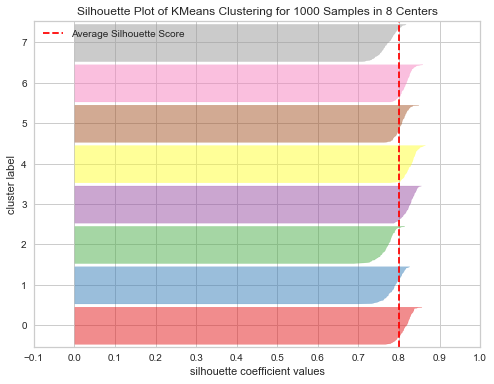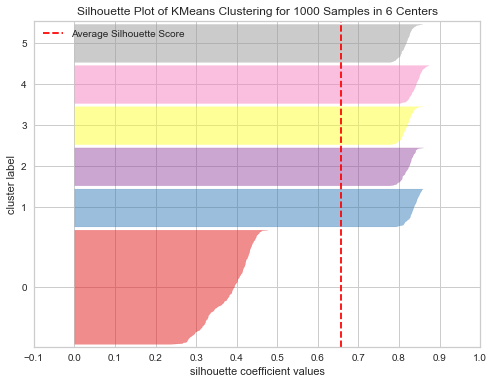# Clustering — We'll Know It When We See It

Posted on October 01, 2018 in articles

During the last year, I've become a core contributor to Yellowbrick, an open source Python project that extends the scikit-learn API with a new core object, the Visualizer. As the project has grown, they've developed several different types of visualizers:

• data visualizers — visualize instances relative to the model space
• score visualizers — visualize model performance
• model selection visualizers — compare multiple models against each other

While this may sound confusing at first, the visualizers are grouped according to the type of analysis that they are best suited for, which brings us (finally!) to the actual topic of this post—clustering visualizers! The clustering visualizers work by wrapping scikit-learn estimators via their fit() method, and once the model is trained, calling show() to display the plot.

Unsupervised models can be particularly difficult to evaluate since we lack any labeled data. Not to be indelicate, but lacking ground truth for our dataset, what always comes to mind is a quote by former Supreme Court Justice Potter Stewart, "I know it when I see it." However, for this walk-though we will actually have the advantage of knowing the ideal number of clusters, since I'll use scikit-learn's make_blobs() function to create a sample two-dimensional dataset with 8 random groups of points. This may seem like cheating at first; however, I found this helpful when I was first learning, since it gave me a basis of comparison from which to start from.

In :
%matplotlib inline

In :
import pandas as pd
import matplotlib.pyplot as plt

from sklearn.cluster import KMeans
from sklearn.datasets import make_blobs

from yellowbrick.cluster import KElbowVisualizer, SilhouetteVisualizer

plt.rcParams["figure.figsize"] = (8,6)

In :
# Generate synthetic dataset with 8 blobs
X, y = make_blobs(n_samples=1000, n_features=15, centers=8, random_state=42)


## Elbow Method¶

K-Means is a simple unsupervised machine learning algorithm that groups data into the number $K$ of clusters specified by the user, even if it is not the optimal number of clusters for the dataset.

Yellowbrick's KElbowVisualizer implements the “elbow” method of selecting the optimal number of clusters by fitting the K-Means model with a range of values for $K$. If the line chart looks like an arm, then the “elbow” (the point of inflection on the curve) is a good indication that the underlying model fits best at that point.

In the following example, the KElbowVisualizer fits the model for a range of $K$ values from 4 to 11, which is set by the parameter k=(4,12). When the model is fit with 8 clusters we can see an "elbow" in the graph, which in this case we know to be the optimal number since we created our synthetic dataset with 8 clusters of points.

In :
# Instantiate the clustering model and visualizer
model = KMeans()
visualizer = KElbowVisualizer(model, k=(4,12))

visualizer.fit(X)         # Fit the data to the visualizer
visualizer.show();        # Draw/show the dataBy default, the scoring parameter metric is set to distortion, which computes the sum of squared distances from each point to its assigned center. However, two other metrics can also be used with the KElbowVisualizersilhouette and calinski_harabasz. The silhouette score is the mean silhouette coefficient for all samples, while the calinski_harabasz score computes the ratio of dispersion between and within clusters.

The KElbowVisualizer also displays the amount of time to fit the model per $K$, which can be hidden by setting timings=False. In the following example, I'll use the calinski_harabasz score and hide the time to fit the model.

In :
# Instantiate the clustering model and visualizer
model = KMeans()
visualizer = KElbowVisualizer(model, k=(4,12), metric='calinski_harabasz', timings=False)

visualizer.fit(X)         # Fit the data to the visualizer
visualizer.show();        # Draw/show the dataIt is important to remember that the Elbow method does not work well if the data is not very clustered. In this case, you might see a smooth curve and the optimal value of $K$ will be unclear.

## Silhouette Visualizer¶

Silhouette analysis can be used to evaluate the density and separation between clusters. The score is calculated by averaging the silhouette coefficient for each sample, which is computed as the difference between the average intra-cluster distance and the mean nearest-cluster distance for each sample, normalized by the maximum value. This produces a score between -1 and +1, where scores near +1 indicate high separation and scores near -1 indicate that the samples may have been assigned to the wrong cluster.

The SilhouetteVisualizer displays the silhouette coefficient for each sample on a per-cluster basis, allowing users to visualize the density and separation of the clusters. This is particularly useful for determining cluster imbalance or for selecting a value for $K$ by comparing multiple visualizers.

Since we created the sample dataset for these examples, we already know that the data points are grouped into 8 clusters. So for the first SilhouetteVisualizer example, we'll set $K$ to 8 in order to show how the plot looks when using the optimal value of $K$.

Notice that graph contains homogeneous and long silhouettes. In addition, the vertical red-dotted line on the plot indicates the average silhouette score for all observations.

In :
# Instantiate the clustering model and visualizer
model = KMeans(8)
visualizer = SilhouetteVisualizer(model)

visualizer.fit(X)         # Fit the data to the visualizer
visualizer.show();        # Draw/show the dataFor the next example, let's see what happens when using a non-optimal value for $K$, in this case, 6.

Now we see that the width of clusters 1 and 2 have both increased and their silhouette coefficient scores have dropped. This occurs because the width of each silhouette is proportional to the number of samples assigned to the cluster. The model is trying to fit our data into a smaller than optimal number of clusters, making two of the clusters larger (wider) but much less cohesive (as we can see from their below-average scores).

In :
# Instantiate the clustering model and visualizer
model = KMeans(6)
visualizer = SilhouetteVisualizer(model)

visualizer.fit(X)         # Fit the data to the visualizer
visualizer.show();        # Draw/show the dataThat's it for now, but if you're interested in learning more about Yellowbrick, I've also posted a walk-through of Yellowbrick's regression visualizers and will hopefully finish similar posts for the classification, text, feature and model selection visualizers over the next couple of months.